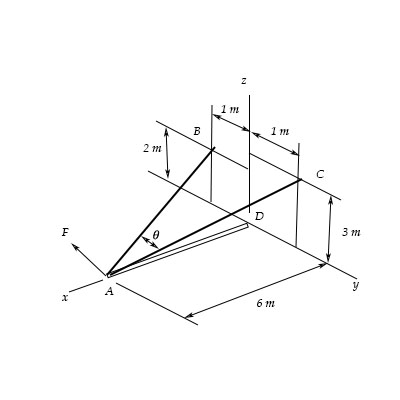# Determine the magnitude of the projected component of the force F={450i-300j+550k} N acting along...

## Question:

Determine the magnitude of the projected component of the force {eq}F={450i-300j+550k} N {/eq} acting along the cable {eq}BA. {/eq}## Vectors:

Vectors in Cartesian Coordinate system can have three different components: the ith, jth, and kth components.

The magnitude of a vector {eq}V {/eq} can be calculated by adding the components

{eq}V = \sqrt{x^2 + y^2 + z^2} {/eq}

Where x is the x component, y is the y component, and z is the z component.

The projected component of F is

{eq}|F| = \sqrt{x^2 + y^2 + z^2} \\ |F| = \sqrt{450^2 + (-300)^2 + 550^2} \\ |F| = 771N {/eq}

The magnitude of the vector in terms of unit m is

{eq}V = \sqrt{x^2 + y^2 + z^2} \\ V = \sqrt{6^2 + 1^2 + 2^2} \\ V = 6.4m {/eq}